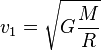Remove from favorites

# Orbital velocity formula calculator

Orbital velocity formula calculator allows you to calculate orbital velocity of an object, at which it move to orbit of a planet in the solar system, or other massive body with defined mass and radius.

## Select a planet or enter mass and radius

Planet:
Orbital velocity (first cosmic velocity) is the minimum velocity, required to object, like artificial satellite, to keep it in orbit of planet.where M - mass of the planet, R - radius of the planet, G - Universal Gravitational Constant = 6.67408 x 10-11Н*м2/kg2